#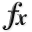N-D Test Functions W¶

class go_benchmark.Watson(dimensions=6)

Watson test objective function.

This class defines the Watson global optimization problem. This is a unimodal minimization problem defined as follows: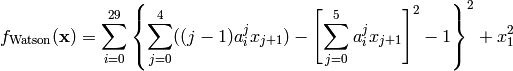Where, in this exercise,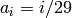.

Here,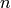represents the number of dimensions andfor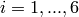.

Global optimum: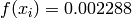for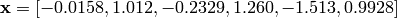class go_benchmark.Wavy(dimensions=2)

W / Wavy test objective function.

This class defines the W / Wavy global optimization problem. This is a multimodal minimization problem defined as follows:Where, in this exercise,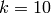. The number of local minima isandfor odd and evenrespectively.

Here,represents the number of dimensions and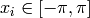for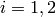.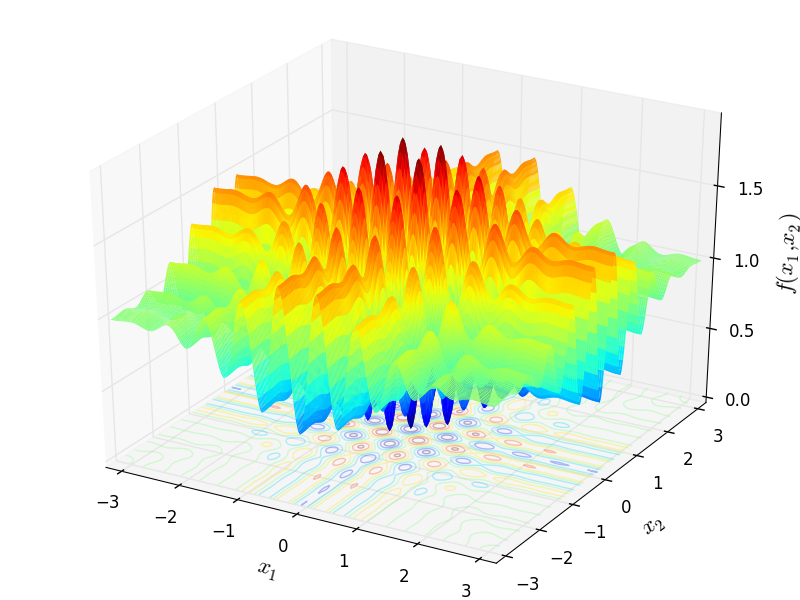Two-dimensional W / Wavy function

Global optimum: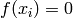for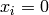forWayburn and Seader 1 test objective function.

This class defines the Wayburn and Seader 1 global optimization problem. This is a unimodal minimization problem defined as follows: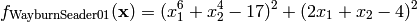Here,represents the number of dimensions andfor.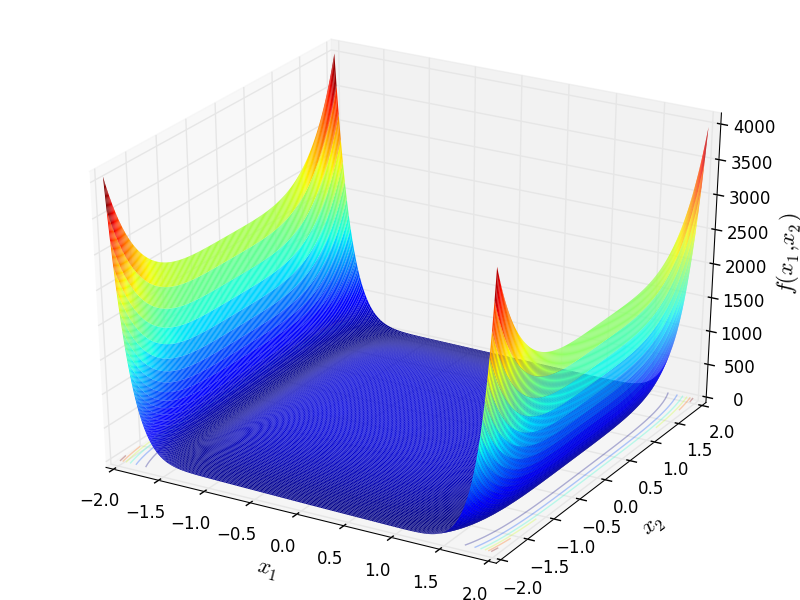Two-dimensional Wayburn and Seader 1 function

Global optimum:for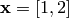Wayburn and Seader 2 test objective function.

This class defines the Wayburn and Seader 2 global optimization problem. This is a unimodal minimization problem defined as follows:Here,represents the number of dimensions and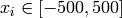for.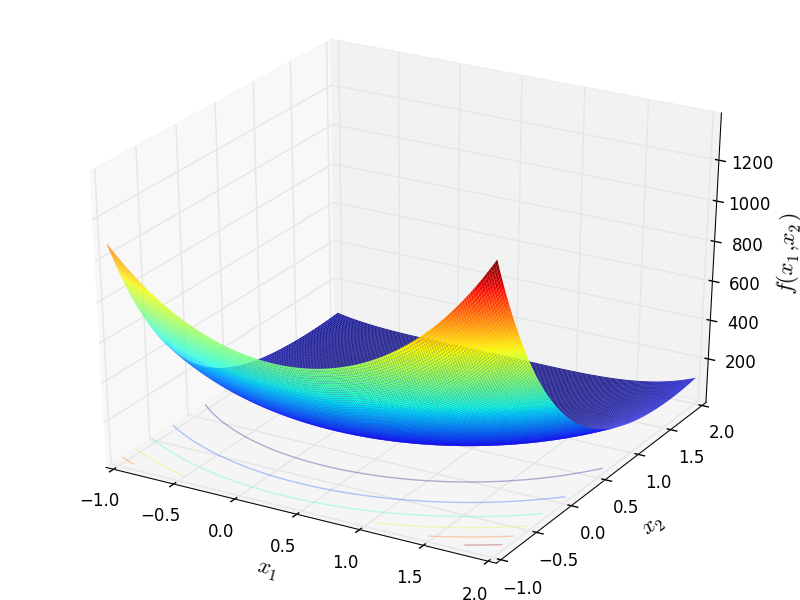Two-dimensional Wayburn and Seader 2 function

Global optimum:forclass go_benchmark.Weierstrass(dimensions=2)

Weierstrass test objective function.

This class defines the Weierstrass global optimization problem. This is a multimodal minimization problem defined as follows: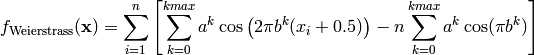Where, in this exercise,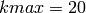,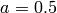and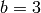.

Here,represents the number of dimensions andfor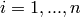.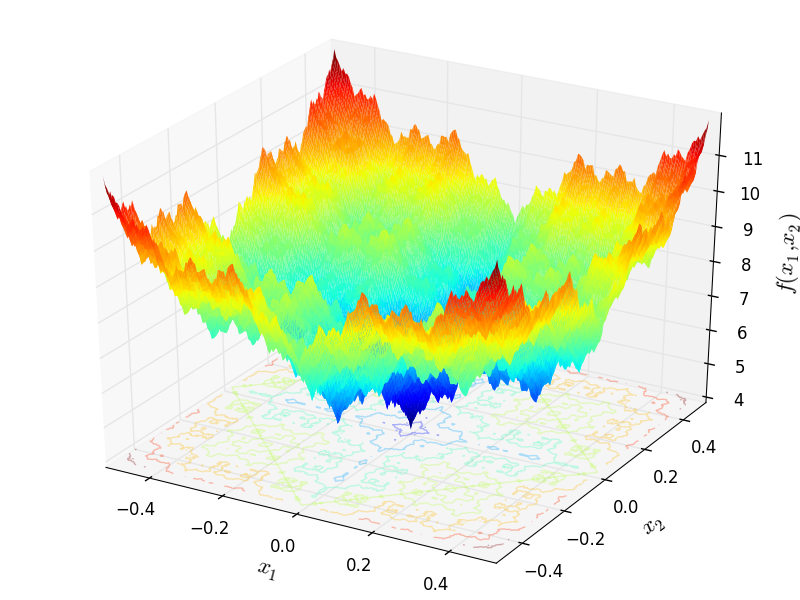Two-dimensional Weierstrass function

Global optimum: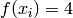forforclass go_benchmark.Whitley(dimensions=2)

Whitley test objective function.

This class defines the Whitley global optimization problem. This is a multimodal minimization problem defined as follows: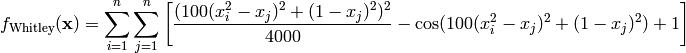Here,represents the number of dimensions and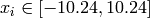for.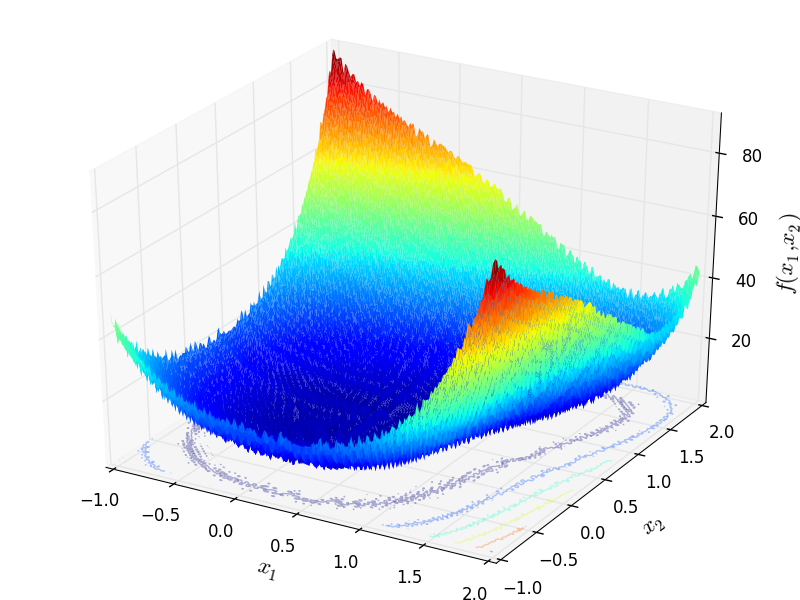Two-dimensional Whitley function

Global optimum:for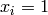forclass go_benchmark.Wolfe(dimensions=3)

Wolfe test objective function.

This class defines the Wolfe global optimization problem. This is a multimodal minimization problem defined as follows: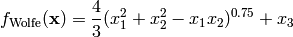Here,represents the number of dimensions andfor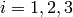.

Global optimum:forfor#### Previous topic

N-D Test Functions V

#### Next topic

N-D Test Functions X